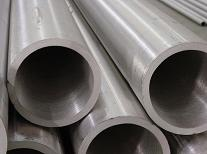# 精密钢管产品名称： 精密钢管 规　　格： 精密钢管 产品备注： 精密钢管
产品说明：
 规格 规格 规格 规格 规格 规格 规格 规格 规格 Φ4×0.5 Φ16×0.5 Φ26×1.8 Φ35×9.0 Φ48×5.0 Φ70×2.8 Φ90×10 Φ130×14 Φ190×14 Φ4×0.8 Φ16×0.8 Φ26×2.0 Φ35×10 Φ48×5.5 Φ70×3.0 Φ90×12 Φ130×16 Φ190×16 Φ4×1.0 Φ16×1.0 Φ26×2.2 Φ38×0.5 Φ48×6.0 Φ70×3.5 Φ90×14 Φ130×18 Φ190×18 Φ4×1.2 Φ16×1.2 Φ26×2.5 Φ38×0.8 Φ48×7.0 Φ70×4.0 Φ90×16 Φ140×2.5 Φ190×20 Φ5×0.5 Φ16×1.5 Φ26×2.8 Φ38×1.0 Φ48×8.0 Φ70×4.5 Φ95×2.0 Φ140×2.8 Φ190×22 Φ5×0.8 Φ16×1.8 Φ26×3.0 Φ38×1.2 Φ48×9.0 Φ70×5.0 Φ95×2.2 Φ140×3.0 Φ200×3.5 Φ5×1.0 Φ16×2.0 Φ26×3.5 Φ38×1.5 Φ48×10 Φ70×5.5 Φ95×2.5 Φ140×3.5 Φ200×4.0 Φ5×1.2 Φ16×2.2 Φ26×4.0 Φ38×1.8 Φ50×1.0 Φ70×6.0 Φ95×2.8 Φ140×4.0 Φ200×4.5 Φ6×0.5 Φ16×2.5 Φ26×4.5 Φ38×2.0 Φ50×1.2 Φ70×7.0 Φ95×3.0 Φ140×4.5 Φ200×5.0 Φ6×0.8 Φ16×2.8 Φ26×5.0 Φ38×2.2 Φ50×1.5 Φ70×8.0 Φ95×3.5 Φ140×5.0 Φ200×5.5 Φ6×1.0 Φ16×3.0 Φ26×5.5 Φ38×2.5 Φ50×1.8 Φ70×9.0 Φ95×4.0 Φ140×5.5 Φ200×6.0 Φ6×1.2 Φ16×3.5 Φ26×6.0 Φ38×2.8 Φ50×2.0 Φ70×10 Φ95×4.5 Φ140×6.0 Φ200×7.0 Φ6×1.5 Φ16×4.0 Φ26×7.0 Φ38×3.0 Φ50×2.2 Φ70×12 Φ95×5.0 Φ140×7.0 Φ200×8.0 Φ6×1.8 Φ16×4.5 Φ26×8.0 Φ38×3.5 Φ50×2.5 Φ70×14 Φ95×5.5 Φ140×8.0 Φ200×9.0 Φ6×2.0 Φ16×5.0 Φ28×0.5 Φ38×4.0 Φ50×2.8 Φ75×1.0 Φ95×6.0 Φ140×9.0 Φ200×10 Φ7×0.5 Φ16×5.5 Φ28×0.8 Φ38×4.5 Φ50×3.0 Φ75×1.2 Φ95×7.0 Φ140×10 Φ200×12 Φ7×0.8 Φ16×6.0 Φ28×1.0 Φ38×5.0 Φ50×3.5 Φ75×1.5 Φ95×8.0 Φ140×12 Φ200×14 Φ7×1.0 Φ18×0.5 Φ28×1.2 Φ38×5.5 Φ50×4.0 Φ75×1.8 Φ95×9.0 Φ140×14 Φ200×16 Φ7×1.2 Φ18×0.8 Φ28×1.5 Φ38×6.0 Φ50×4.5 Φ75×2.0 Φ95×10 Φ140×16 Φ200×18 Φ7×1.5 Φ18×1.0 Φ28×1.8 Φ38×7.0 Φ50×5.0 Φ75×2.2 Φ95×12 Φ140×18 Φ200×20 Φ7×1.8 Φ18×1.2 Φ28×2.0 Φ38×8.0 Φ50×5.5 Φ75×2.5 Φ95×14 Φ150×3.0 Φ200×22 Φ7×2.0 Φ18×1.5 Φ28×2.2 Φ38×9.0 Φ50×6.0 Φ75×2.8 Φ95×16 Φ150×3.5 ​ Φ8×0.5 Φ18×1.8 Φ28×2.5 Φ38×10 Φ50×7.0 Φ75×3.0 Φ95×18 Φ150×4.0 Φ8×0.8 Φ18×2.0 Φ28×2.8 Φ40×0.5 Φ50×8.0 Φ75×3.5 Φ100×2.0 Φ150×4.5 Φ8×1.0 Φ18×2.2 Φ28×3.0 Φ40×0.8 Φ50×9.0 Φ75×4.0 Φ100×2.2 Φ150×5.0 Φ8×1.2 Φ18×2.5 Φ28×3.5 Φ40×1.0 Φ50×10 Φ75×4.5 Φ100×2.5 Φ150×5.5 Φ8×1.5 Φ18×2.8 Φ28×4.0 Φ40×1.2 Φ55×1.0 Φ75×5.0 Φ100×2.8 Φ150×6.0 Φ8×1.8 Φ18×3.0 Φ28×4.5 Φ40×1.5 Φ55×1.2 Φ75×5.5 Φ100×3.0 Φ150×7.0 Φ8×2.0 Φ18×3.5 Φ28×5.0 Φ40×1.8 Φ55×1.5 Φ75×6.0 Φ100×3.5 Φ150×8.0 Φ8×2.2 Φ18×4.0 Φ28×5.5 Φ40×2.0 Φ55×1.8 Φ75×7.0 Φ100×4.0 Φ150×9.0 Φ8×2.5 Φ18×4.5 Φ28×6.0 Φ40×2.2 Φ55×2.0 Φ75×8.0 Φ100×4.5 Φ150×10 Φ9×0.5 Φ18×5.0 Φ28×7.0 Φ40×2.5 Φ55×2.2 Φ75×9.0 Φ100×5.0 Φ150×12 Φ9×0.8 Φ18×5.5 Φ28×8.0 Φ40×2.8 Φ55×2.5 Φ75×10 Φ100×5.5 Φ150×14 Φ9×1.0 Φ18×6.0 Φ30×0.5 Φ40×3.0 Φ55×2.8 Φ75×12 Φ100×6.0 Φ150×16 Φ9×1.2 Φ20×0.5 Φ30×0.8 Φ40×3.5 Φ55×3.0 Φ75×14 Φ100×7.0 Φ150×18 Φ9×1.5 Φ20×0.8 Φ30×1.0 Φ40×4.0 Φ55×3.5 Φ75×16 Φ100×8.0 Φ150×20 Φ9×1.8 Φ20×1.0 Φ30×1.2 Φ40×4.5 Φ55×4.0 Φ80×1.0 Φ100×9.0 Φ160×3.0 Φ9×2.0 Φ20×1.2 Φ30×1.5 Φ40×5.0 Φ55×4.5 Φ80×1.2 Φ100×10 Φ160×3.5 Φ9×2.2 Φ20×1.5 Φ30×1.8 Φ40×5.5 Φ55×5.0 Φ80×1.5 Φ100×12 Φ160×4.0 Φ9×2.5 Φ20×1.8 Φ30×2.0 Φ40×6.0 Φ55×5.5 Φ80×1.8 Φ100×14 Φ160×4.5 Φ9×2.8 Φ20×2.0 Φ30×2.2 Φ40×7.0 Φ55×6.0 Φ80×2.0 Φ100×16 Φ160×5.0 Φ10×0.5 Φ20×2.2 Φ30×2.5 Φ40×8.0 Φ55×7.0 Φ80×2.2 Φ100×18 Φ160×5.5 Φ10×0.8 Φ20×2.5 Φ30×2.8 Φ40×9.0 Φ55×8.0 Φ80×2.5 Φ110×2.0 Φ160×6.0 Φ10×1.0 Φ20×2.8 Φ30×3.0 Φ40×10 Φ55×9.0 Φ80×2.8 Φ110×2.2 Φ160×7.0 Φ10×1.2 Φ20×3.0 Φ30×3.5 Φ42×1.0 Φ55×10 Φ80×3.0 Φ110×2.5 Φ160×8.0 Φ10×1.5 Φ20×3.5 Φ30×4.0 Φ42×1.2 Φ55×12 Φ80×3.5 Φ110×2.8 Φ160×9.0 Φ10×1.8 Φ20×4.0 Φ30×4.5 Φ42×1.5 Φ60×1.0 Φ80×4.0 Φ110×3.0 Φ160×10 Φ10×2.0 Φ20×4.5 Φ30×5.0 Φ42×1.8 Φ60×1.2 Φ80×4.5 Φ110×3.5 Φ160×12 Φ10×2.2 Φ20×5.0 Φ30×5.5 Φ42×2.0 Φ60×1.5 Φ80×5.0 Φ110×4.0 Φ160×14 Φ10×2.5 Φ20×5.5 Φ30×6.0 Φ42×2.2 Φ60×1.8 Φ80×5.5 Φ110×4.5 Φ160×16 Φ10×2.8 Φ20×6.0 Φ30×7.0 Φ42×2.5 Φ60×2.0 Φ80×6.0 Φ110×5.0 Φ160×18 Φ10×3.0 Φ20×7.0 Φ30×8.0 Φ42×2.8 Φ60×2.2 Φ80×7.0 Φ110×5.5 Φ160×20 Φ12×0.5 Φ22×0.5 Φ30×9.0 Φ42×3.0 Φ60×2.5 Φ80×8.0 Φ110×6.0 Φ170×3.0 Φ12×0.8 Φ22×0.8 Φ30×10 Φ42×3.5 Φ60×2.8 Φ80×9.0 Φ110×7.0 Φ170×3.5 Φ12×1.0 Φ22×1.0 Φ32×0.5 Φ42×4.0 Φ60×3.0 Φ80×10 Φ110×8.0 Φ170×4.0 Φ12×1.2 Φ22×1.2 Φ32×0.8 Φ42×4.5 Φ60×3.5 Φ80×12 Φ110×9.0 Φ170×4.5 Φ12×1.5 Φ22×1.5 Φ32×1.0 Φ42×5.0 Φ60×4.0 Φ80×14 Φ110×10 Φ170×5.0 Φ12×1.8 Φ22×1.8 Φ32×1.2 Φ42×5.5 Φ60×4.5 Φ80×16 Φ110×12 Φ170×5.5 Φ12×2.0 Φ22×2.0 Φ32×1.5 Φ42×6.0 Φ60×5.0 Φ85×1.5 Φ110×14 Φ170×6.0 Φ12×2.2 Φ22×2.2 Φ32×1.8 Φ42×7.0 Φ60×5.5 Φ85×1.8 Φ110×16 Φ170×7.0 Φ12×2.5 Φ22×2.5 Φ32×2.0 Φ42×8.0 Φ60×6.0 Φ85×2.0 Φ110×18 Φ170×8.0 Φ12×2.8 Φ22×2.8 Φ32×2.2 Φ42×9.0 Φ60×7.0 Φ85×2.2 Φ120×2.0 Φ170×9.0 Φ12×3.0 Φ22×3.0 Φ32×2.5 Φ42×10 Φ60×8.0 Φ85×2.5 Φ120×2.2 Φ170×10 Φ12×3.5 Φ22×3.5 Φ32×2.8 Φ45×1.0 Φ60×9.0 Φ85×2.8 Φ120×2.5 Φ170×12 Φ12×4.0 Φ22×4.0 Φ32×3.0 Φ45×1.2 Φ60×10 Φ85×3.0 Φ120×2.8 Φ170×14 Φ14×0.5 Φ22×4.5 Φ32×3.5 Φ45×1.5 Φ60×12 Φ85×3.5 Φ120×3.0 Φ170×16 Φ14×0.8 Φ22×5.0 Φ32×4.0 Φ45×1.8 Φ65×1.0 Φ85×4.0 Φ120×3.5 Φ170×18 Φ14×1.0 Φ22×5.5 Φ32×4.5 Φ45×2.0 Φ65×1.2 Φ85×4.5 Φ120×4.0 Φ170×20 Φ14×1.2 Φ22×6.0 Φ32×5.0 Φ45×2.2 Φ65×1.5 Φ85×5.0 Φ120×4.5 Φ180×3.5 Φ14×1.5 Φ22×7.0 Φ32×5.5 Φ45×2.5 Φ65×1.8 Φ85×5.5 Φ120×5.0 Φ180×4.0 Φ14×1.8 Φ25×0.5 Φ32×6.0 Φ45×2.8 Φ65×2.0 Φ85×6.0 Φ120×5.5 Φ180×4.5 Φ14×2.0 Φ25×0.8 Φ32×7.0 Φ45×3.0 Φ65×2.2 Φ85×7.0 Φ120×6.0 Φ180×5.0 Φ14×2.2 Φ25×1.0 Φ32×8.0 Φ45×3.5 Φ65×2.5 Φ85×8.0 Φ120×7.0 Φ180×5.5 Φ14×2.5 Φ25×1.2 Φ32×9.0 Φ45×4.0 Φ65×2.8 Φ85×9.0 Φ120×8.0 Φ180×6.0 Φ14×2.8 Φ25×1.5 Φ32×10 Φ45×4.5 Φ65×3.0 Φ85×10 Φ120×9.0 Φ180×7.0 Φ14×3.0 Φ25×1.8 Φ35×0.5 Φ45×5.0 Φ65×3.5 Φ85×12 Φ120×10 Φ180×8.0 Φ14×3.5 Φ25×2.0 Φ35×0.8 Φ45×5.5 Φ65×4.0 Φ85×14 Φ120×12 Φ180×9.0 Φ14×4.0 Φ25×2.2 Φ35×1.0 Φ45×6.0 Φ65×4.5 Φ85×16 Φ120×14 Φ180×10 Φ14×4.5 Φ25×2.5 Φ35×1.2 Φ45×7.0 Φ65×5.0 Φ90×1.5 Φ120×16 Φ180×12 Φ15×0.5 Φ25×2.8 Φ35×1.5 Φ45×8.0 Φ65×5.5 Φ90×1.8 Φ120×18 Φ180×14 Φ15×0.8 Φ25×3.0 Φ35×1.8 Φ45×9.0 Φ65×6.0 Φ90×2.0 Φ130×2.5 Φ180×16 Φ15×1.0 Φ25×3.5 Φ35×2.0 Φ45×10 Φ65×7.0 Φ90×2.2 Φ130×2.8 Φ180×18 Φ15×1.2 Φ25×4.0 Φ35×2.2 Φ48×1.0 Φ65×8.0 Φ90×2.5 Φ130×3.0 Φ180×20 Φ15×1.5 Φ25×4.5 Φ35×2.5 Φ48×1.2 Φ65×9.0 Φ90×2.8 Φ130×3.5 Φ190×3.5 Φ15×1.8 Φ25×5.0 Φ35×2.8 Φ48×1.5 Φ65×10 Φ90×3.0 Φ130×4.0 Φ190×4.0 Φ15×2.0 Φ25×5.5 Φ35×3.0 Φ48×1.8 Φ65×12 Φ90×3.5 Φ130×4.5 Φ190×4.5 Φ15×2.2 Φ25×6.0 Φ35×3.5 Φ48×2.0 Φ65×14 Φ90×4.0 Φ130×5.0 Φ190×5.0 Φ15×2.5 Φ25×7.0 Φ35×4.0 Φ48×2.2 Φ70×1.0 Φ90×4.5 Φ130×5.5 Φ190×5.5 Φ15×2.8 Φ25×8.0 Φ35×4.5 Φ48×2.5 Φ70×1.2 Φ90×5.0 Φ130×6.0 Φ190×6.0 Φ15×3.0 Φ26×0.5 Φ35×5.0 Φ48×2.8 Φ70×1.5 Φ90×5.5 Φ130×7.0 Φ190×7.0 Φ15×3.5 Φ26×0.8 Φ35×5.5 Φ48×3.0 Φ70×1.8 Φ90×6.0 Φ130×8.0 Φ190×8.0 Φ15×4.0 Φ26×1.0 Φ34×6.0 Φ48×3.5 Φ70×2.0 Φ90×7.0 Φ130×9.0 Φ190×9.0 Φ15×4.5 Φ26×1.2 Φ35×7.0 Φ48×4.0 Φ70×2.2 Φ90×8.0 Φ130×10 Φ190×10 Φ15×5.0 Φ26×1.5 Φ35×8.0 Φ48×4.5 Φ70×2.5 Φ90×9.0 Φ130×12 Φ190×12

﻿